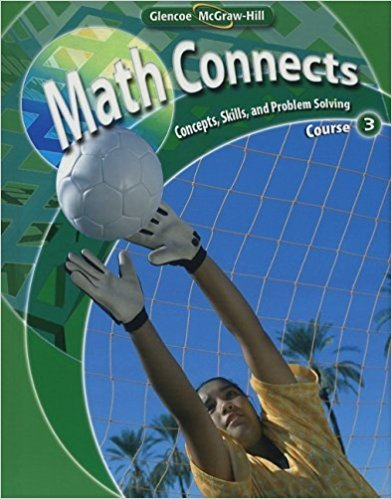×
×

# Solutions for Chapter 3: Math Connects: Concepts, Skills, and Problem Solving Course 3 0th Edition## Full solutions for Math Connects: Concepts, Skills, and Problem Solving Course 3 | 0th Edition

ISBN: 9780078740503Solutions for Chapter 3

Solutions for Chapter 3
4 5 0 286 Reviews
12
2
##### ISBN: 9780078740503

Since 9 problems in chapter 3 have been answered, more than 1126 students have viewed full step-by-step solutions from this chapter. Math Connects: Concepts, Skills, and Problem Solving Course 3 was written by and is associated to the ISBN: 9780078740503. This textbook survival guide was created for the textbook: Math Connects: Concepts, Skills, and Problem Solving Course 3, edition: 0. This expansive textbook survival guide covers the following chapters and their solutions. Chapter 3 includes 9 full step-by-step solutions.

Key Math Terms and definitions covered in this textbook
• Basis for V.

Independent vectors VI, ... , v d whose linear combinations give each vector in V as v = CIVI + ... + CdVd. V has many bases, each basis gives unique c's. A vector space has many bases!

• Cholesky factorization

A = CTC = (L.J]))(L.J]))T for positive definite A.

• Commuting matrices AB = BA.

If diagonalizable, they share n eigenvectors.

• Diagonalization

A = S-1 AS. A = eigenvalue matrix and S = eigenvector matrix of A. A must have n independent eigenvectors to make S invertible. All Ak = SA k S-I.

• Fast Fourier Transform (FFT).

A factorization of the Fourier matrix Fn into e = log2 n matrices Si times a permutation. Each Si needs only nl2 multiplications, so Fnx and Fn-1c can be computed with ne/2 multiplications. Revolutionary.

• Fourier matrix F.

Entries Fjk = e21Cijk/n give orthogonal columns FT F = nI. Then y = Fe is the (inverse) Discrete Fourier Transform Y j = L cke21Cijk/n.

• Fundamental Theorem.

The nullspace N (A) and row space C (AT) are orthogonal complements in Rn(perpendicular from Ax = 0 with dimensions rand n - r). Applied to AT, the column space C(A) is the orthogonal complement of N(AT) in Rm.

• Gauss-Jordan method.

Invert A by row operations on [A I] to reach [I A-I].

• Hermitian matrix A H = AT = A.

Complex analog a j i = aU of a symmetric matrix.

• Kirchhoff's Laws.

Current Law: net current (in minus out) is zero at each node. Voltage Law: Potential differences (voltage drops) add to zero around any closed loop.

• Kronecker product (tensor product) A ® B.

Blocks aij B, eigenvalues Ap(A)Aq(B).

• Lucas numbers

Ln = 2,J, 3, 4, ... satisfy Ln = L n- l +Ln- 2 = A1 +A~, with AI, A2 = (1 ± -/5)/2 from the Fibonacci matrix U~]' Compare Lo = 2 with Fo = O.

• Markov matrix M.

All mij > 0 and each column sum is 1. Largest eigenvalue A = 1. If mij > 0, the columns of Mk approach the steady state eigenvector M s = s > O.

• Nilpotent matrix N.

Some power of N is the zero matrix, N k = o. The only eigenvalue is A = 0 (repeated n times). Examples: triangular matrices with zero diagonal.

• Normal equation AT Ax = ATb.

Gives the least squares solution to Ax = b if A has full rank n (independent columns). The equation says that (columns of A)·(b - Ax) = o.

• Orthogonal matrix Q.

Square matrix with orthonormal columns, so QT = Q-l. Preserves length and angles, IIQxll = IIxll and (QX)T(Qy) = xTy. AlllAI = 1, with orthogonal eigenvectors. Examples: Rotation, reflection, permutation.

• Orthogonal subspaces.

Every v in V is orthogonal to every w in W.

• Spanning set.

Combinations of VI, ... ,Vm fill the space. The columns of A span C (A)!

• Spectral Theorem A = QAQT.

Real symmetric A has real A'S and orthonormal q's.

• Vector space V.

Set of vectors such that all combinations cv + d w remain within V. Eight required rules are given in Section 3.1 for scalars c, d and vectors v, w.

×